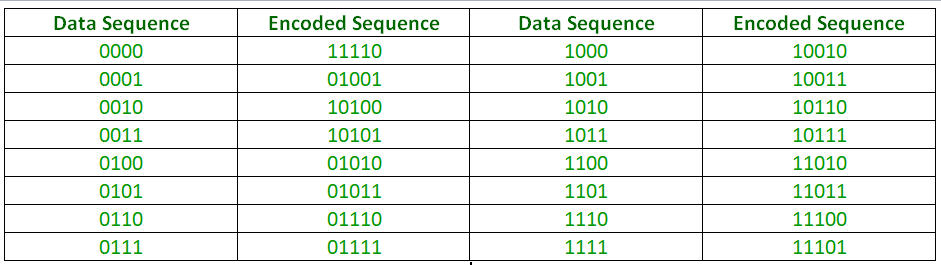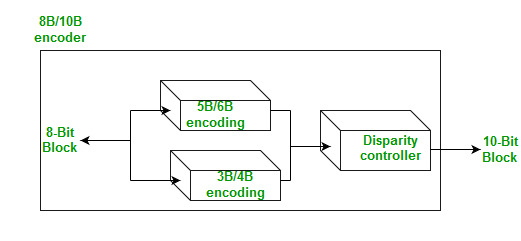# Block Coding in Digital Electronics

• Last Updated : 25 Nov, 2019

Conversion of Digital Data to Digital Signal involves three techniques:

1. Line Coding
2. Block Coding
3. Scrambling

Out of which Line coding is always needed, block coding and scrambling may or may not be needed.

Attention reader! Don’t stop learning now. Get hold of all the important CS Theory concepts for SDE interviews with the CS Theory Course at a student-friendly price and become industry ready.

Block coding helps in error detection and re-transmission of the signal. It is normally referred to as mB/nB coding as it replaces each m-bit data group with an n-bit data group (where n>m). Thus, its adds extra bits (redundancy bits) which helps in synchronization at receiver’s and sender’s end and also providing some kind of error detecting capability.

It normally involves three steps: division, substitution, and combination. In the division step,a sequence of bits is divided into groups of m-bits. In the substitution step, we substitute an m-bit group for an n-bit group. Finally, the n-bit groups are combined together to form a stream which has more bits than the original bits.

Examples of mB/nB coding:

4B/5B (four binary/five binary ) –
This coding scheme is used in combination with NRZ-I. The problem with NRZ-I was that it has a synchronization problem for long sequences of zeros. So, to overcome it we substitute the bit stream from 4-bit to 5-bit data group before encoding it with NRZ-I. So that it does not have a long stream of zeros. The block-coded stream does not have more than three consecutive zeros (see encoding table).At the receiver, the NRZ-I encoded digital signal is first decoded into a stream of bits and then decoded again to remove the redundancy bits.

Drawback – Though 4B/5B encoding solves the problem of synchronization,it increases the signal rate of NRZ-L.Moreover,it does not solve the DC component problem of NRZ-L.

8B/10B (eight binary/ten binary) –
This encoding is similar to 4B/5B encoding except that a group of 8 bits of data is now substituted by a 10-bit code and it provides greater error detection capability than 4B/5B.
It is actually a combination of 5B/6B and 3B/4B encoding.The most five significant bits of a 10-bit block is fed into the 5B/6B encoder; the least 3 significant bits is fed into a 3B/4B encoder. The split is done to simplify the mapping table.A group of 8 bits can have 2^8 different combinations while a group of 10 bits can have 2^10 different combinations. This means that there are 2^10-2^8=768 redundant groups that are not used for 8B/10B encoding and can be used for error detection and disparity check.

Thus, this technique is better than 4B/5B because of better error-checking capability and better synchronization.

Reference-
Data Communications and Networking By Behrouz A.Forouzan(Book)

My Personal Notes arrow_drop_up